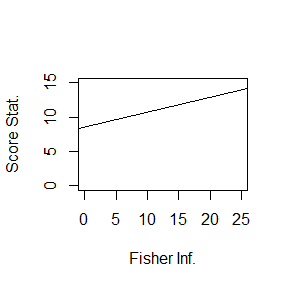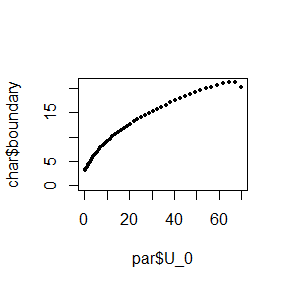# Design and Analysis of Clinical Trials with Fully Flexible Adaptive Sample Size Determination

#### 2018-09-20

In this vignettes, an application of a locally and a globally efficient adaptive sample determination to a confirmatory randomized clinical trial is illustrated.

# Clinical Trial Example

This trial evaluated whether oral adjuvant chemotherapy with tegaful and uracil (UFT) and leucovorin (LV) reduces the recurrence after resection of liver metastasis from colorectal carcinoma as compared with no adjuvant therapy in Japan (UFT/LV trial) (Hasegawa et al. PLoS One 2016;11:e0162400.). The null hypothesis $$log(HR) = 0$$ was tested with the one-sided significance level of 0.025. The minimum of clinically important effect size was hypothesized as HR = 0.65. The test statistic was a stratified log-rank score. Suppose that four interim analyses and one final analysis were planned to be performed but when to perform was not fixed in advance.

The result of the interim analyses were as follows. * Fisher information at analyses: (5.67, 9.18, 14.71, 20.02) * Score statistic = (3.40, 4.35, 7.75, 11.11)

## Locally efficient adaptive design

The initial working test (SPRT) is prepared as a basis of conditional error function. Its stopping boundary is $$-\log(\alpha) / \rho + 1 / 2 \rho t$$, where the significance level $$\alpha = 0.025$$ and the minimum of clinically important effect size $$\rho = -log(0.65)$$ will be substituted and $$t$$ is the Fisher information. This stopping boundary is depicted below.

# Working test: sequential probability ratio test (SPRT)
plot(1, 1, type="n", xlim=c(0, 25), ylim=c(0, 15), xlab="Fisher Inf.", ylab = "Score Stat.")
alpha <- 0.025
rho <- -log(0.65)
abline(-log(alpha) / rho, 1/2 * rho)The four interim analyses can be performed by the function adaptive_analysis_norm_local. Designating FALSE to the argument final_analysis indicates that the latest analysis is not the final, i.e., the overall significance level must not be exhausted at this time.

# Final interim analysis
interim_analysis_4 <- adaptive_analysis_norm_local(
overall_sig_level = 0.025,
min_effect_size = -log(0.65),
times = c(5.67, 9.18, 14.71, 20.02),
stats = c(3.40, 4.35, 7.75, 11.11),
final_analysis = FALSE
)

The result is summarized as follows:

# Summary
print( with(interim_analysis_4, data.frame(analysis=0:par$analyses, time=par$times,
intercept=char$intercept, stat=par$stats, boundary=char$boundary, pr_cond_err=char$cond_type_I_err, reject_H0=char$rej_H0)) ) #> analysis time intercept stat boundary pr_cond_err reject_H0 #> 1 0 0.00 8.563198 0.00 8.563198 0.02500000 FALSE #> 2 1 5.67 8.562666 3.40 9.783935 0.06392209 FALSE #> 3 2 9.18 8.562085 4.35 10.539378 0.06951043 FALSE #> 4 3 14.71 8.551346 7.75 11.719755 0.18084726 FALSE #> 5 4 20.02 8.456860 11.11 12.768997 0.48935479 FALSE At the forth (final) interim analysis, the null hypothesis is not rejected. Then, the maximum sample size (here, the maximum Fisher information level) is calculated. The alternative hypothesis for which an adequate level of power will be ensured can be determined arbitrarily referring to all available data including the interim results but not correlates of future data. Here, the maximum likelihood estimate $$11.11 / 20.02$$ at the forth interim analysis is chosen as the alternative hypothesis. The maximum information level to obtaine the marginal power of 0.75 can be calculated by the function sample_size_norm_local. # Sample size calculation sample_size_norm_local( overall_sig_level = 0.025, min_effect_size = -log(0.65), effect_size = 11.11 / 20.02, # needs not be MLE time = 20.02, target_power = 0.75, sample_size = TRUE ) #>  24.44479 Finally, suppose that the final analysis is performed at $$t = 24.44$$. The same function used at interim analyses, adaptive_analysis_norm_local, can be used with setting final_analysis = TRUE. # Final analysis final_analysis <- adaptive_analysis_norm_local( overall_sig_level = 0.025, min_effect_size = -log(0.65), times = c(5.67, 9.18, 14.71, 20.02, 24.44), stats = c(3.40, 4.35, 7.75, 11.11, 14.84), final_analysis = TRUE ) Again, the result is summarized as: # Summary print( with(final_analysis, data.frame(analysis=0:par$analyses, time=par$times, intercept=char$intercept, stat=par$stats, boundary=char$boundary,
pr_cond_err=char$cond_type_I_err, reject_H0=char$rej_H0)) )
#>   analysis  time intercept  stat  boundary pr_cond_err reject_H0
#> 1        0  0.00  8.563198  0.00  8.563198  0.02500000     FALSE
#> 2        1  5.67  8.562666  3.40  9.783935  0.06392209     FALSE
#> 3        2  9.18  8.562085  4.35 10.539378  0.06951043     FALSE
#> 4        3 14.71  8.551346  7.75 11.719755  0.18084726     FALSE
#> 5        4 20.02  8.456860 11.11 12.768997  0.48935479     FALSE
#> 6        5 24.44        NA 14.84 11.166106  1.00000000      TRUE

As indicated at the final row, the null hypothesis is rejected.

## Globally efficient adaptive design

Globally efficient adaptive design can be performed in a similar way by using the functions for globally efficient functions.

The initial working test, a group sequential design with 50 analyses, is prepared as a basis of conditional error function. Its stopping boundary can be constructed by the function work_test_norm_global.

init_work_test <- work_test_norm_global(min_effect_size = -log(0.65), cost_type_1_err = 0)

Here, cost_type_1_err = 0 means the value of loss caused by erroneous rejection of the null hypothesis is calculated to make the constructed working test have exactly the type I error probability of $$\alpha$$. The default value of cost_type_1_err is 0 and thus can be omitted. The boundary of the working test just constructed is displayed by the next code.

with(init_work_test, plot(par$U_0, char$boundary, xlim=range(0, par$U_0), ylim=range(0, char$boundary[-1]), pch=16, cex=0.5) )The four interim analyses can be performed by the function adaptive_analysis_norm_global. Designating FALSE to the argument final_analysis indicates that the latest analysis is not the final, i.e., the overall significance level must not be exhausted at this time.

# Final interim analysis
interim_analysis_4 <- adaptive_analysis_norm_global(
initial_test = init_work_test,
times = c(5.67, 9.18, 14.71, 20.02),
stats = c(3.40, 4.35, 7.75, 11.11),
final_analysis = FALSE,
estimate = FALSE
)

The result is:

# Summary
print( with(interim_analysis_4, data.frame(analysis=0:par$analyses, time=par$times,
cost=char$cost0, stat=par$stats, boundary=char$boundary, pr_cond_err=char$cond_type_I_err,
reject_H0=char$rej_H0)) ) #> analysis time cost stat boundary pr_cond_err reject_H0 #> 1 0 0.00 1683.458 0.00 Inf 0.02500000 FALSE #> 2 1 5.67 1555.020 3.40 7.004168 0.06006569 FALSE #> 3 2 9.18 1545.278 4.35 8.690863 0.06007655 FALSE #> 4 3 14.71 1528.397 7.75 10.724362 0.15229716 FALSE #> 5 4 20.02 1471.727 11.11 12.239176 0.39095697 FALSE At the forth (final) interim analysis, the null hypothesis is not rejected. Then, the maximum Fisher information level is calculated. The maximum likelihood estimate $$11.11 / 20.02$$ at the forth interim analysis is chosen as the alternative hypothesis, though this is not compelling. The maximum information level to obtaine the marginal power of $$0.75$$ can be calculated by the function sample_size_norm_global. # Sample size calculation sample_size_norm_global( initial_test = init_work_test, effect_size = 11.11 / 20.02, # needs not be MLE time = 20.02, target_power = 0.75, sample_size = TRUE ) #>  25.88426 Finally, suppose that the final analysis is performed at $$t = 25.88$$. The same function used at interim analyses can be used, with setting final_analysis = TRUE. # Final analysis final_analysis <- adaptive_analysis_norm_global( initial_test = init_work_test, times = c(5.67, 9.18, 14.71, 20.02, 25.88), stats = c(3.40, 4.35, 7.75, 11.11, 14.84), costs = interim_analysis_4$char$cost0[-1], # Omited element is for time = 0 final_analysis = TRUE, estimate = FALSE ) # Summary print( with(final_analysis, data.frame(analysis=0:par$analyses, time=par$times, cost=char$cost0, stat=par$stats, boundary=char$boundary, pr_cond_err=char$cond_type_I_err, reject_H0=char$rej_H0)) )
#>   analysis  time     cost  stat  boundary pr_cond_err reject_H0
#> 1        0  0.00 1683.458  0.00       Inf  0.02500000     FALSE
#> 2        1  5.67 1555.020  3.40  7.004168  0.06006569     FALSE
#> 3        2  9.18 1545.278  4.35  8.690863  0.06007655     FALSE
#> 4        3 14.71 1528.397  7.75 10.724362  0.15229716     FALSE
#> 5        4 20.02 1471.727 11.11 12.239176  0.39095697     FALSE
#> 6        5 25.88       NA 14.84 11.780124  1.00000000      TRUE

As indicated by the final row, the null hypothesis is rejected.

Note that, if estimate = TRUE, additionally exact P-value, median unbiased estimate, and confidence limits can be calculated. These results will be extracted by:

# Estimte (P-value, median unbiased estimate, and confidence limits)
print( final_analysis\$est )